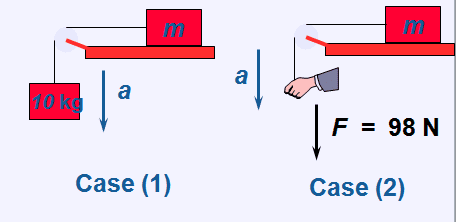# Basic forces problem: which has a higher acceleration?

• JSmithDawg

## Homework Statement

In which situation does block m experience a larger acceleration? In case 1, the 10kg mass is hanging from a rope and falling. In case 2, a hand is providing a constant downward force. Assume the rope is massless.F=ma

## The Attempt at a Solution

The force applied to m in case 1 is 98N. Gravity is acting on both 'm' and 10kg, but since 'm' is on top of a table (which provides a normal force), it's going to have no effect on the system's acceleration. Knowing F=ma, F=10kg(9.8 m/s^2), F=98N. Thus, the force in case 1 is equal to the force in case 2. However, the online quiz I did told me that case 2 has the higher acceleration. How is this possible if the downward force is the same in both situations?

What's the total mass being accelerated in each case?

You could try drawing "the hand of gravity" in case 1.

The total mass being accelerated for case one is 10kg+m, and for case two it's m. But isn't the 'm' insignificant when looking at how much force is applied to each system? Wait, but it might be significant when determining acceleration. F=ma, so 98N=11kg(a) vs. 98N = 1kg(a). That makes sense that case two will accelerate faster! Thank you for hinting at the law of inertia!